# Test: Frequency Response

## 10 Questions MCQ Test Topicwise Question Bank for Electronics Engineering | Test: Frequency Response

Description
Attempt Test: Frequency Response | 10 questions in 30 minutes | Mock test for Electronics and Communication Engineering (ECE) preparation | Free important questions MCQ to study Topicwise Question Bank for Electronics Engineering for Electronics and Communication Engineering (ECE) Exam | Download free PDF with solutions
QUESTION: 1

### The mid frequency gain of the amplifier circuit shown below with high value of beta of the BJT is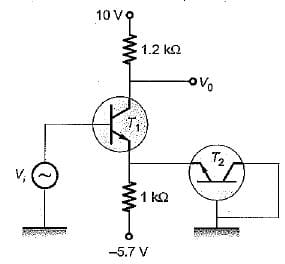Solution:

Applying KVL, we get: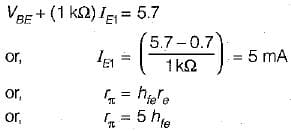Now,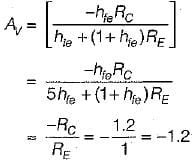or, this diagram is CE unbypass amplifier so,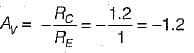QUESTION: 2

Solution:
QUESTION: 3

### An npn BJT has gm = 38 mΩ/V, Cμ = 10-14 F, Cπ = 4 x 10-13 F and DC current gain, β0 = 90. For this transistor fT and fβ are respectively

Solution: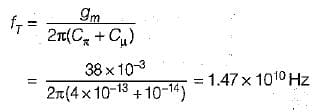and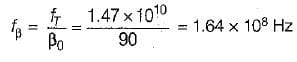QUESTION: 4

G iven for an FET, gm = - 9 .5 m A /volt, total capacitance = 500 pF. For a voltage gain of -30, the bandwidth will be

Solution:
QUESTION: 5

The drain gate capacitance of a junction FET is 2 pF. Assuming a common source voltage gain of 20, what is the input capacitance due to miller effect?

Solution:
QUESTION: 6

Assertion (A): In the high frequency model of FET, the voltage amplification drops drastically with increase in frequency.
Reason (R): The high frequency model is identical with the low frequency model except that the capacitances between various terminals (gate, source and drain) is added.

Solution:

The high frequency mode! of FET is shown below.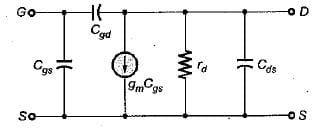Because of the internal capacitances (Cgd, Cgs and Cds), feedback exists between the output and input circuits of the FET in its high frequency model and hence the volta g e amplification drops drastically with the increase in frequency. Moreover, there is no feedback from output to input in the FET small signal (low frequency) model.
Flence, both assertion and reason are correct and reason is the correct explanation of assertion.

QUESTION: 7

For a single stage CE amplifier, gm = 50 mA/V, Ce= 1 pF and Cc = 0.2 pF. The frequency at which the short circuit common-emitter current gain attains unit magnitude is given by

Solution:

The frequency at which the short circuit common- emitter current gain attains unit magnitude is given by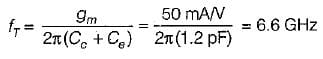QUESTION: 8

When the load resistance is zero (short circuit) in a common-emitter configuration, the current flowing from the base to the collector node through the collector capacitor Cc, is small because

Solution:

Ce can be as smai| as 1 pF, and Cc as small as 0.2 pF.
Thus, Cis larger than Cc

QUESTION: 9

Consider the following statements:
1. For finding the transistor behaviour at high frequency it is necessary to examine the diffusion mechanism.
2. At low frequency it is assumed that the transistor responds instantly to changes of input voltage or current.
3. The hybrid-jr model is valid under dynamic conditions when the rate of change of \/BEis small enough so that the base incremental current/^ is small compared with the collector incremental current/^
4. The parameter fTis a frequency characteristic of a transistor.

Which of the above statements are correct?

Solution:
• The mechanism of the transport of charge carriers from emitter to collector is essentially one of diffusion. Hence, for finding the transistor behaviour at high frequency it is n e ce ssa ry to exam ine the d iffu sio n mechanism. Hence, statement-1 is correct.
• Statement-2 is also correct.
• Statement-3 is also correct.
• The frequency at which the short-circuit common-emitter current gain attains unit magnitude is represented by fT Thus, Statement - 4 is also correct.
QUESTION: 10

A modern bipolar transistor can have Ce = 1 pF. If gm = 50 mA/V, the fT of a common emitter amplifier will be

Solution: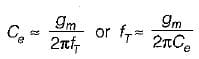or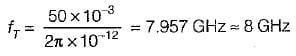Use Code STAYHOME200 and get INR 200 additional OFF Use Coupon Code